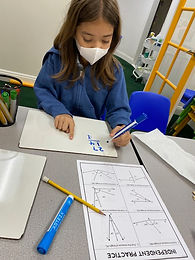## Ms. Dawn

### Target 1​

###### Lesson Type:

Continuation

Geometry

:

Shape

Classify 2D and 3D figures based on the basic properties of shapes.

###### 1:

Define each type of triangle (scalene, isosceles, equilateral, obtuse, acute, and right).

###### 2:

Understand that a triangle can be describe by two categories (i.e., right scalene, acute isosceles, etc.).

4th

###### Vocabulary:

Triangle, Scalene, Isosceles, Equilateral, Acute, Obtuse, Right, Congruent, Classify, Properties, Angles

Activities:

1. Revisited the definitions of scalene, isosceles, equilateral, acute, obtuse, and right. Also, addressed what congruent meant.

2. Students then continued their poster on classifying triangle based on their properties. They needed to look at the triangle and identify the angle name, angle description, side name, and side description.

3. After completing the poster, students played a game called, "Monster Munch," which allowed students to display their knowledge of the vocabulary words (Scalene, Isosceles, Equilateral, Right)### Home Exploration

###### Guiding Questions:## Absent Students:

### Target 2

:

###### 1:

Understand that angles can be composed or decomposed, using addition and subtraction.

4th

###### Vocabulary:

Composed, Decomposed, Angles, Straight angle, Right angle, Missing angle

Activities:

Students needed to find the measurement of a variety of missing angles. It started off simple with all angles given, so they needed to add it altogether to get the complete angle. Then, they were given the total angle measurement and one of the angles. They needed to find the other missing angle to make the statement true. Last, they needed to find the mystery angle based on prior knowledge attributes of what a straight or right angle represents.### Home Exploration

###### Guiding Questions:### Target 3

:

###### Vocabulary:

Activities:### Home Exploration